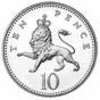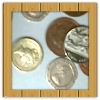#### You may also like### Coin Tossing Games

You and I play a game involving successive throws of a fair coin. Suppose I pick HH and you pick TH. The coin is thrown repeatedly until we see either two heads in a row (I win) or a tail followed by a head (you win). What is the probability that you win?### Win or Lose?

A gambler bets half the money in his pocket on the toss of a coin, winning an equal amount for a head and losing his money if the result is a tail. After 2n plays he has won exactly n times. Has he more money than he started with?### Thank Your Lucky Stars

A counter is placed in the bottom right hand corner of a grid. You toss a coin and move the star according to the following rules: ... What is the probability that you end up in the top left-hand corner of the grid?

# Same Number!

##### Age 14 to 16Challenge Level

Imagine you are in a class of thirty students. The teacher asks everyone to secretly write down a whole number between 1 and 225.
Do you think it's likely or unlikely that everyone's number will be different?

You could try this out in your class a few times, or experiment with this simulation:

How often was everyone's number different?
Are you surprised by this?

Let's try to explain what happens:
Imagine the teacher asks students to read out their numbers one at a time.
What is the probability that the second student reads out a number that is different to the one read out by the first student?

If the first two students have read out different numbers, what is the chance that the third student will read out another new number?
What is the probability that the first three students all read out different numbers?

If the first three students have all read out different numbers, what is the chance that the fourth student will read out another new number?
What is the probability that the first four students all read out different numbers?
...

What is the probability that the whole class of thirty students read out different numbers?
What is the probability that at least two students have written the same number?

*************************************************

There is a famous mathematical problem called the Birthday Problem:

How many people do you need in a room so that the chance that there
will be at least two people with the same birthday is greater than 50%?

One way to solve this is to imagine that people enter the room one at a time, and that each new person doesn't share a birthday with anyone already there...